## 3.3.2 A 3D Kinematic Chain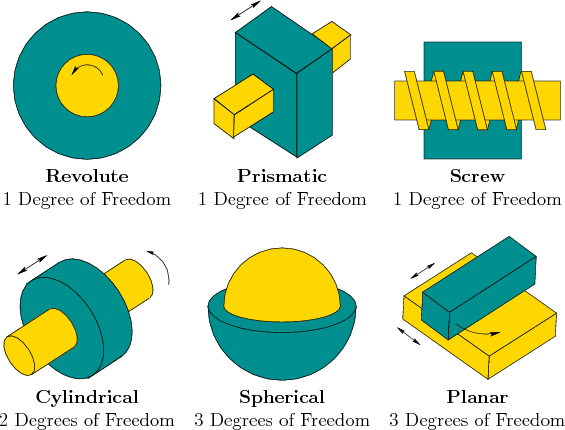As for a single rigid body, the 3D case is significantly more complicated than the 2D case due to 3D rotations. Also, several more types of joints are possible, as shown in Figure 3.12. Nevertheless, the main ideas from the transformations of 2D kinematic chains extend to the 3D case. The following steps from Section 3.3.1 will be recycled here:

1. The body frame must be carefully placed for each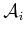.
2. Based on joint relationships, several parameters are measured.
3. The parameters define a homogeneous transformation matrix,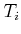.
4. The location in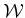of any point in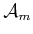is given by applying the matrix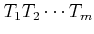.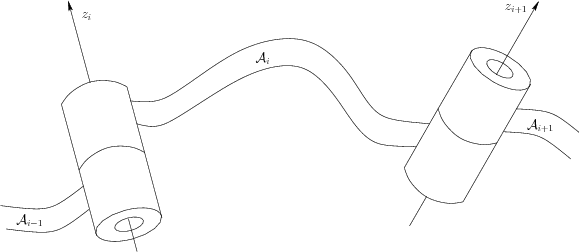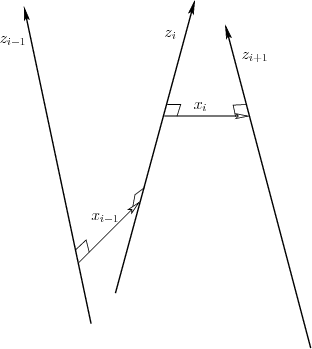Consider a kinematic chain of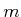links in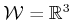, in which eachfor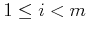is attached to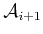by a revolute joint. Each link can be a complicated, rigid body as shown in Figure 3.13. For the 2D problem, the coordinate frames were based on the points of attachment. For the 3D problem, it is convenient to use the axis of rotation of each revolute joint (this is equivalent to the point of attachment for the 2D case). The axes of rotation will generally be skew lines in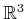, as shown in Figure 3.14. Let the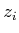-axis be the axis of rotation for the revolute joint that holdsto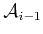. Between each pair of axes in succession, let the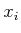-axis join the closest pair of points between the- and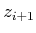-axes, with the origin on the-axis and the direction pointing towards the nearest point of the-axis. This axis is uniquely defined if the- and-axes are not parallel. The recommended body frame for eachwill be given with respect to the- and-axes, which are shown in Figure 3.14. Assuming a right-handed coordinate system, the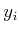-axis points away from us in Figure 3.14. In the transformations that will appear shortly, the coordinate frame given by,, andwill be most convenient for defining the model for. It might not always appear convenient because the origin of the frame may even lie outside of, but the resulting transformation matrices will be easy to understand.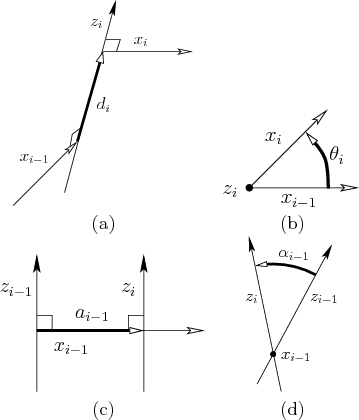In Section 3.3.1, eachwas defined in terms of two parameters,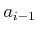and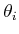. For the 3D case, four parameters will be defined: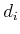,,, and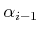. These are referred to as Denavit-Hartenberg (DH) parameters . The definition of each parameter is indicated in Figure 3.15. Figure 3.15a shows the definition of. Note that the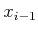- and-axes contact the-axis at two different places. Letdenote signed distance between these points of contact. If the-axis is above the-axis along the-axis, thenis positive; otherwise,is negative. The parameteris the angle between the- and-axes, which corresponds to the rotation about the-axis that moves the-axis to coincide with the-axis. The parameter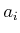is the distance between the- and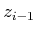-axes; recall these are generally skew lines in. The parameteris the angle between the- and-axes.

Subsections
Steven M LaValle 2012-04-20